Maths Course in English

# Ratio and Proportion Math Formulas and Tricks

Ratio and Proportion Math Formulas and Tricks in English: In this page, you will learn about Ratio and Proportion Formulas and Tricks, Problems, Short Tricks in English. This topic is very important for every competitive exams preparation. So before proceeding for any exam read these short tricks and explanations carefully about Ration and Proportion Formulas and Short Tricks with explanation.

## Formulas of Ratio and Proportion

Ratio is the spoken language of arithmetic. It is the language with which we relate quantities of the same kind. 15 people, for example, are more than 5 people. We can express that by saying how much more, that is, 10 more, or by saying how many times more: Three times more. When we say that 15 people are three times more than 5 people, that is called their ratio.

• The ratio of two terms ‘x’ and ‘y’ is denoted by x: y.
• In general, the ratio of a number x to a number y is defined as the quotient of the numbers x and y.
• The numerator of the ratio is called the antecedent (x) and the denominator is called the consequent (y) of the ratio.

### Properties of Ratio

a : b = m a : m b, where m is a constant

a : b : c = A : B : C is equivalent to,

This is an important property and has to be used in ratio of three things.

Ifthen,This property is called Componendo

For example: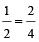So,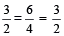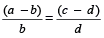This property is called Dividendo

For example:So,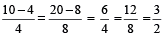This property is called Componendo and Dividendo

For example:So,Ifthen,= each of the individual ratio

For example:Therefore,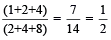If A > B thenWhere A, B and C are natural numbers

For example: 3 > 2, then[ as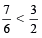]

If A < B then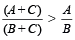Where A, B and C are natural numbers.

For example: 2 < 3, then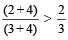[ as]

### To divide a given quantity into a given ratio

Suppose any given quantity a, is to be divided in the ratio m : n.

Let one part of the given quantity be x then the other part will be a – x.

∴or nx = ma – mx

or (m + n) x = ma

∴ one part isand the other part will beExample 1: Divide 70 in the ratio 3 : 7

Solution: Let one part be x, then the other part = 70 – x

∴or 7x = 210 – 3x

or x = 21 and 70 – x = 49

Hence the two required parts of 70 are 21 and 49.

In any 2-dimensional figures, if the corresponding sides are in the ratio x : y, then their areas are in the ratio x² : y².

Example 2: The ratio of the radius of two circles is 2 : 5. Find the ratio of their areas.

Solution: Ratio of their areas = 2² : 5² = 4 : 25

In any two 3-dimensional figures, if the corresponding sides are in the ratio x : y, then their volumes are in the ratio x³ : y³. If the ratio between two numbers is a : b and

if each number is increased by x, the ratio becomes c : d. Then

Sum of the two numbers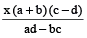Difference of the two numbersTwo numbers are given as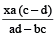andExample 3: The ratio between two numbers is 3 : 4. If each number be increased by 2, the ratio becomes 7 : 9. Find the numbers.

Solution:

Numbers are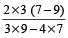andor 12 and 16.

If the sum of two numbers is A and their difference is a, then the ratio of numbers is given by A + a : A – a.

Example 4: The sum of two numbers is 60 and their difference is 6. What is the ratio of the two numbers?

Solution: The required ratio of the numbers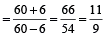or 11 : 9

### Proportion

Proportion is an expression in which two ratios are equal.

For example,

⇒ A : B : : C : D

Here,  A D = B C

Example 5: If 12 : 18 :: x : 24, then find the value of x.

Solution: 12 : 18 : : x : 24

⇒ 12 × 24 = 18 × x

⇒ x == 16

### Type of Proportion

Continued Proportion: If three numbers a, b and c are in continued proportion,then:

We can say that a, b and c are in proportion.

i.e.,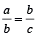⇒ b² = ac

⇒ b =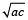Here we can say that a is called first proportion, c is called third proportion and b is called mean proportion.

Direct Proportion: If X is directly proportional to Y, that means any increase or decrease in any of two quantities will have proportionate effect on the other quantity. If X increases then Y will also increase and vice-versa.

Inverse Proportion: If X is inversely proportional to Y, that means any increase or decrease in any of two quantities will have inverse proportionate effect on the other quantity. This means if X increases, then Y decreases and if X decreases then Y increases and vice-versa for Y.

If four quantities are in proportion, the product of the extremes is equal to the product of the means.

Let a, b, c, d be in proportion, thenIf three quantities a, b and c are in continued proportion, then
a : b = b : c

∴ ac = b²

b is called mean proportional.

If three quantities are proportional, then first is to the third is the duplicate ratio of the first is to the second.

If a : b :: b : c then a : c = a² : b²

### To find the mean proportional

Example 6: Find the mean proportional between 3 and 75.

Solution: Let x be the required mean proportional. Then,

3 : x :: x : 75

∴ x =### To find the values of an unknown when four numbers are in proportion

Example 7: What must be added to each of the four numbers 10, 18, 22, 38 so that they become in proportion?

Solution: Let the number to be added to each of the four numbers be x.

By the given condition, we get

(10 + x) : (18 + x) : : (22 + x) : (38 + x)

⇒ (10 + x) (38 + x) = (18 + x) (22 + x)

⇒ 380 + 48x + x² = 396 + 40x + x²

Cancelling x² from both sides, we get

380 + 48x = 396 + 40x

⇒ 48x – 40x = 396 – 380

⇒ 8x = 16

⇒Therefore, 2 should be added to each of the four given numbers.

### To find the fourth proportional

Example 8: Find the fourth proportional to p² – pq + q², p³ + q³, p – q

Solution: Let x be the fourth proportional

∴ (p²– pq + q²) : (p³ + q³) = (p – q) : x

⇒ (p² – pq + q²) × x = (p³ + q³) (p – q)

∴⇒⇒∴ The required fourth proportional is p² – q²

### To find the third proportional

Example 9: Find third proportional to a² – b² and a + b.

Solution: Let x be the required third proportional

Then a² – b² : a + b = a + b : x

∴ (a² – b²) x = (a + b) (a + b)

∴### Using theorem on equal proportion

Example 10: If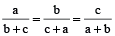, prove that each is equal toor –1.

Solution: We have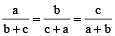Each ratio =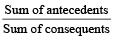[By theorem on equal ratios]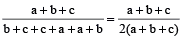=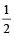ifIf a + b + c = 0, then b + c = –a

∴Similarly,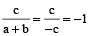Hence each ratio =if= –1 if a + b + c = 0